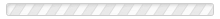Search:

(clear)

• p
• physical constant
The constant c, the speed of light in a vacuum, is an example of a physical constant. It is a constant of nature, always(...) - Read More
• pi
Pi is symbolized π and pronounced like "pie." It is the mathematical symbol for the number 3.14159…, which goes on(...) - Read More
• Pilot Wave Interpretation of quantum mechanics
This interpretation of quantum mechanics is also called the "Bohmian Interpretation." It was originally proposed by(...) - Read More
• probability amplitude
“Probability amplitude” is a term used in quantum physics. It’s a number that appears in Schrodinger’s Wave Equation. Let’s(...) - Read More
• probability wave
Max Born, one of the early quantum physicists in the 1920's and '30s, proposed that between detections, quantum particles(...) - Read More
• q
• QFT
See Quantum Field Theory. - Read More
• quantities of dimension one
For “quantities of dimension one,” see “dimensionless number.” - Read More
• quantum
One definition of “quantum” is: a tiny packet of energy at the atomic level. A quantum is the smallest possible unit of(...) - Read More
• quantum angular momentum
For quantum angular momentum, see angular momentum. - Read More
• quantum decoherence
For quantum decoherence, see  decoherence. - Read More
• quantum entanglement
In quantum physics, if two particles are entangled, their behavior is correlated. In addition, if a physicist obtains(...) - Read More
• Quantum Field Theory
Quantum Field Theory (QFT) is the current theory of how atomic and subatomic particles behave. It is the most up-to-date(...) - Read More
• quantum mechanics
In quantum mechanics, physicists study how tiny particles behave. These include atoms, the components of atoms like(...) - Read More
• quantum number
Electrons have a few handfuls of properties. Four have been selected as the electron’s “quantum numbers.” The quantum(...) - Read More
• quantum physics
For an understanding of quantum physics, see the definition of "quantum mechanics." Often, the two terms are used to mean(...) - Read More
• quantum randomness
Many quantum physicists view quantum mechanics as differing from classical physics in a fundamental way—quantum mechanics is(...) - Read More
• quantum realm
A number of interpretations of quantum mechanics postulate a “quantum realm.” These include the Transactional(...) - Read More
• quantum spin